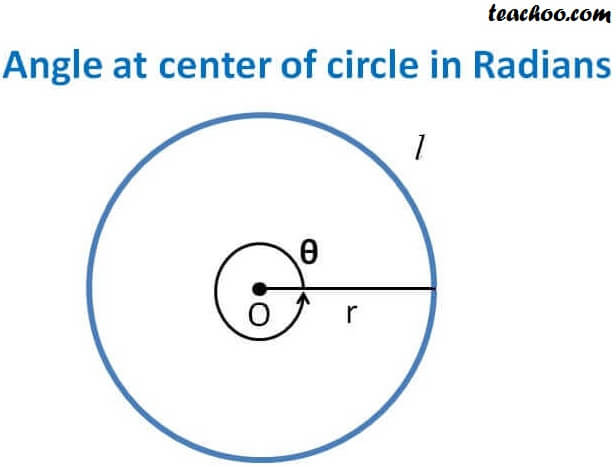Chapter 3 Class 11 Trigonometric Functions
Concept wise

Let us consider a circle of radius r

Let Angle subtended by whole circle at center be θ

Length of arc = Circumference of circle = 2πrNow,

We know that

θ = l/r

θ = 2πr/r

θ = 2π

∴ Angle at center of circle in Radians is 2 π

Learn in your speed, with individual attention - Teachoo Maths 1-on-1 Class

### Transcript

Let us consider a circle of radius r Let Angle subtended by whole circle at center be Length of arc = Circumference of circle = 2 r Now, We know that = l/r = 2 r/r = 2 Angle at center of circle in Radians is 2